Brakes

Drum Brakes

Introduction

The drum brake widely is used on road vehicles and consists of a drum attached to the rotating wheel. The drum has an internal machined cylindrical surface.   Inside the drum and protected from the environment are two shoes lined with friction material which can be pivotted to make a forced contact with the internal cylindrical surface.

Nomenclature
 F = Applied Force (N) P = Brake Power kW T = Torque (Nm) F = Applied Force (N) Ff = Friction Force (N) Fn = Normal force between drum and friction pad (N) μ = Coefficient of Friction. b = Band width (m) c = Distance drum pivot to centre of drum(m) MFf = Friction moment about shoe pivot (Nm) MFn = Normal Force moment about shoe pivot (Nm) n = Rotational Speed (RPM) p = pressure for friction surface (N/m2) p max = Maximum pressure for friction surface(N/m2) θ = Brake shoe contact angle r = Radius of brake wheel)(m)

Theory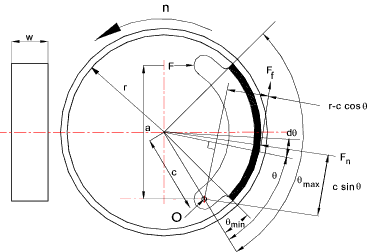The pressure distribution evaluation for the brake shoe assumes that the shoe arm is rigid and the pressure is directly related to the distance from the pivot point.  The pressure is proportional to c sin θ or p = k sin θ where k is some constant.

For a specific brake material the maximum pressure = p max.  Therefore..

k = p max /sin θ max... and...

p = sin θ(p max /sin θ max)
The value of minimum θ is usually set to above 10 0 and the maximum pressure when the value of sin θ is 90 0. above this angle the pressure reduces.   Therefore if the maximum value of θ is less than 90 0 then sin θ max = sin θ. if the maximum angle is greater than 900 then sin θ max = sin 90 0

Torque Capacity for Brake Shoe

The torque capacity of a brake shoe is obtained by integrating the product of the frictional force and the drum radius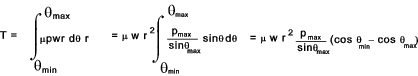Actuating force required for Brake Shoe

The actuating force is calculated by taking moments about the brake shoe pivot point.    MFn is the moment due to the normal force between the shoe and the drum.  MFf is the moment due to the friction force.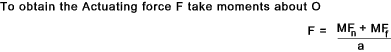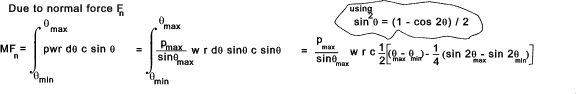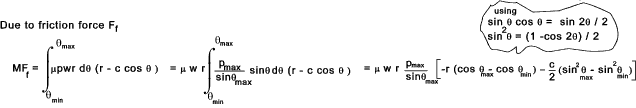The motion of the drum as shown result in an ACW friction moment and the normal reaction force of the drum also results in an ACW moment as the two moments are the same self locking cannot occur.   If the rotation is reversed and the friction moment is CW then the resulting actuating force will be reduced and self-locking is possible.

Opposite handed brake show

The opposite handed brake shoe as shown below results in the friction moment being of opposite to the normal force moment and the brake system is to some extent self energising with a possibility of self locking
To obtain the maximum torque for each brake shoe...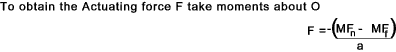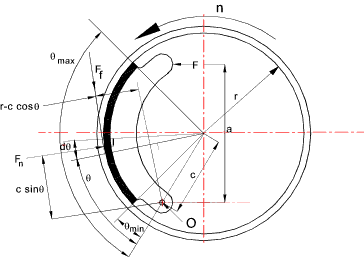Links to Brake Design Introduction to Braking systems A very detailed document reviewing all aspects of car braking systems Theory of Machines -Friction devices ..Various calculators Clutches and Brakes ..A large detailed downloadable book containing lots of relevant information

Brakes